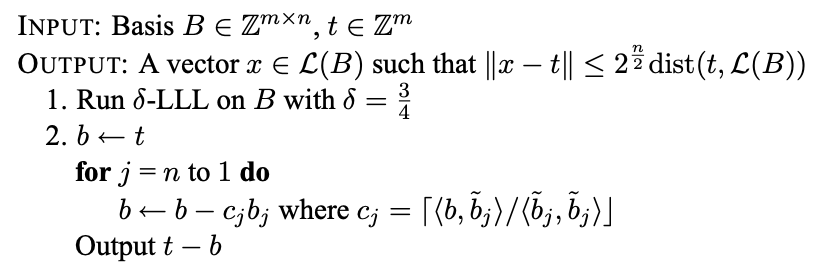# CVP¶

CVP is a particularly important issue in Lattice-based cryptography.

The basic definition of the problem is as follows: Given a set of bases and vectors $\mathbf{v}$ for $L$, find the nearest vector to $\mathbf{v}$ on $L$.

## Algorithms¶

### Babai's nearest plane algorithm¶

The algorithm inputs a set of lattice $L$ (rank is $n$) base $B$ and a target vector $\mathbf{t}$ to output an approximate solution to the CVP problem.

• The approximation factor is $\gamma = 2^{\frac{n}{2}}$

Specific algorithm:• where $c_j$ is the rounding of the coefficients in the Gram-schmidt orthogonalization, which is the rounding of $proj_{b_{j}}(b)$.

For the personal understanding of the second step of the algorithm: find a linear combination closest to $\mathbf{t}$ in the base $B$ after the lattice basis and the orthogonalization.

### Babai’s Rounding Technique¶

This algorithm is a variant of Babai&#39;s nearest plane algorithm.

The steps can be expressed as:

N = rank(B), w = target

- B' = LLL(B)

- Find a linear combination [l_0, ... l_N] such that w = sum(l_i * b'_i).

* (b'_i is the i-th vector in the LLL-reduced basis B')

- Round each l_i to it's closest integer l'_i.

- Result v = sum(l'_i * b'_i)


### Hidden number problem¶

The definition of HNP is as follows:

Given the prime $p$, many $t \in \mathbb{F}_p$ and each corresponding $MSB_{l,p}(\alpha t)$, find the corresponding $\alpha$.

• $MSB_{l,p}(x)$ means any integer $u that satisfies $\lvert (x \mod p) - u \rvert \le \frac{p}{2^{l+1}}$$, which is approximately $l$ most significant digits of $x \mod p$.

According to the description in Reference 3, when $l \approx \log^{\frac{1}{2}}{p}$, the following algorithm can solve HNP:

We can turn this problem into a CVP problem on the lattice generated by the matrix:

$\left[ \begin{matrix} p & 0 & \dots & 0 & 0 \\ 0 & p & \ddots & \vdots & \vdots \\ \vdots & \ddots & \ddots & 0 & \vdots \\ 0 & 0 & \dots & p & 0 \\ t_1 & t_2 & \dots & t_{n} & \frac{1}{2^{l+1}} \end{matrix} \right]$

We need to find the nearest vector from $\mathbf{u}=(u_1, u_2, \dots, u_{n}, 0)$ on the lattice, so here we can use Babai&#39;s nearest plane algorithm. Finally we can get a set of vectors $\mathbf{v}=(\alpha \cdot t_1 \mod p, \alpha \cdot t_2 \mod p, \dots, \frac{\alpha}{2^{l+1} })$, which calculates $\alpha$.

### BCTF 2018 - guess_number¶

The topic provides server-side code:

import random, sys

from flag import FLAG

import gmpy2

def msb(k, x, p):

delta = p &gt;&gt; (k + 1)
ui = random.randint (x-delta, x + delta)
return ui

def main():

p = gmpy2.next_prime(2**160)

for _ in range(5):

alpha = random.randint(1, p - 1)

# print(alpha)

t = []

u = []
k = 10
for i in range(22):

t.append(random.randint(1, p - 1))

u.append(msb(k, alpha * t[i] % p, p))

print(str(t))

print (p (u))
guess = raw_input('Input your guess number: ')

guess = int(guess)

if guess != alpha:

exit(0)

if __name__ == "__main__":

main()

print(FLAG)


As you can see, the program performs a total of 5 rounds. In each round, the program generates a random $\alpha$ and 22 random $t_i$. For each $t_i$, the program will take $u_i = MSB_{10,p}(\alpha\cdot{t_i\mod{p}})$ and send it to the client. We need to calculate the corresponding $\alpha$ based on the provided $t_i$ and $u_i$. As you can see, the problem is a typical Hidden number problem, so you can use the above algorithm to solve:

import socket

import ast
import telnetlib

#HOST, PORT = 'localhost', 9999

HOST, PORT = '60.205.223.220', 9999

s = socket.socket()

s.connect((HOST, PORT))

f = s.makefile('rw', 0)

def recv_until(f, delim='\n'):

buf = &#39;&#39;
while not buf.endswith(delim):

return buf

p = 1461501637330902918203684832716283019655932542983

k = 10

def solve_hnp(t, u):

# http://www.isg.rhul.ac.uk/~sdg/igor-slides.pdf

M = Matrix(RationalField(), 23, 23)

for i in xrange(22):
M[i, i] = p

M[22, i] = t[i]

M[22, 22] = 1 / (2 ** (k + 1))

def babai(A, w):

A = A.LLL(delta=0.75)

G = A.gram_schmidt()

t = w

for i in reversed(range(A.nrows())):

c = ((t * G[i]) / (G[i] * G[i])).round()

t -= A[i] * c

return w - t

closest = babai(M, vector(u + ))

return (closest[-1] * (2 ** (k + 1))) % p

for i in xrange(5):

alpha = solve_hnp(t, u)

recv_until(f, 'number: ')

s.send(str(alpha) + '\n')

t = telnetlib.Telnet()

t.sock = s

t.interact()